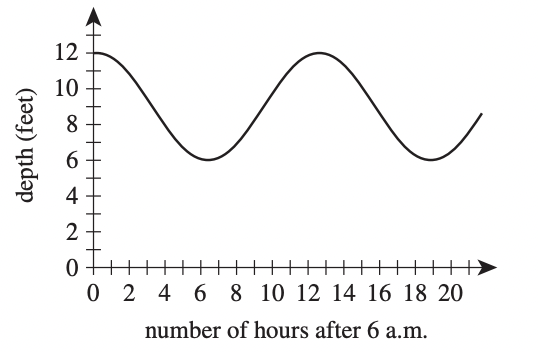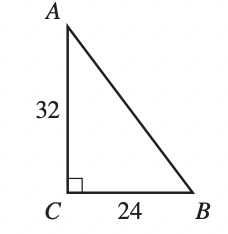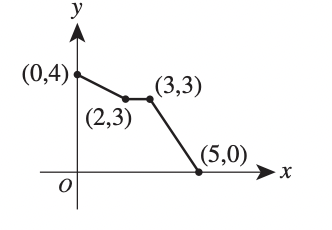# ACT Mathematics: Geometry Quiz (2019-2020 ACT Test)

This is an MCQ-based quiz on the topic of ACT Mathematics: Geometry Quiz (2019-2020 ACT Test).

This set of MCQs helps you brush up on the important mathematics topic and prepare you to dive into skill practice and expand your knowledge to tackle the question and answer it carefully.

Start Quiz

The fluctuation of water depth at a pier is shown in the figure below. One of the following values gives the positive difference, in feet, between the greatest water depth and the least water depth shown in this graph. Which value is it?3

6

12

19

The length of a rectangle is 5 inches longer than the width. The perimeter of the rectangle is 40 inches. What is the width of the rectangle, in inches?

7.5

8

15

16

In the standard (x,y) coordinate plane, what is the slope of the line given by the equation 4x = 7y + 5 ?

-4/7

4/7

7/4

4

The lengths of the 2 legs of right triangle nABC shown below are given in inches. The midpoint of AB ___ is how many inches from A ?16

20

21

28

The graph of y = ⎪x − 6⎪ is in the standard (x,y) coordinate plane. Which of the following transformations, when applied to the graph of y = ⎪x⎪, results in the graph of y = ⎪x − 6⎪ ?

Translation to the right 6 coordinate units

Translation to the left 6 coordinate units

Translation up 6 coordinate units

Translation up 6 coordinate units

A box in the shape of a cube has an interior side length of 18 inches and is used to ship a right circular cylinder with a radius of 6 inches and a height of 12 inches. The interior of the box not occupied by the cylinder is filled with packing material. Which of the following numerical expressions gives the number of cubic inches of the box filled with packing material?

15 - pi

15 + pi

18^3 - pi*6*12*6

pi^3

A room has a rectangular floor that is 15 feet by 21 feet. What is the area of the floor in square yards ?

24

35

36

105

The graph of a function y = f (x) consists of 3 line segments. The graph and the coordinates of the endpoints of the 3 line segments are shown in the standard (x,y) coordinate plane below. What is the area, in square coordinate units, of the region bounded by the graph of y = f(x), the positive y-axis, and the positive x-axis?10

13

14

15

Ray PK → bisects ∠LPM, the measure of ∠LPM is 11x°, and the measure of ∠LPK is (4x + 18)°. What is the measure of ∠KPM ?

12

43

42

66

. In the standard (x,y) coordinate plane, what is the midpoint of the line segment that has endpoints (3,8) and (1,−4) ?

-2, -12

-1, -6

11/2, -3/2

2,2

Quiz/Test Summary
Title: ACT Mathematics: Geometry Quiz (2019-2020 ACT Test)
Questions: 10
Contributed by: